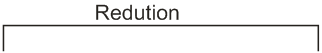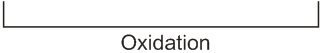# Calculate the standard cell potential (in V) of the cell in which following reaction takes place:Given thatOption 1)x-zOption 2)x-yOption 3)x+2y-3zOption 4)x+y-zGiven

Cell equation :-

(Oxidation)                    (Cathode Reduction)

Std. EMF of given cell reaction:-

(iii-ii)

Put this in eqn (i)

Option 1)

x-z

Option 2)

x-y

Option 3)

x+2y-3z

Option 4)

x+y-z

Exams
Articles
Questions